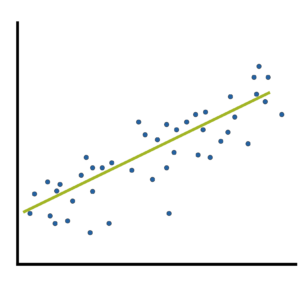# power calculation

### Two Types of Effect Size Statistic: Standardized and Unstandardized

June 26th, 2023 by

Effect size statistics are all the rage these days.

Journal editors are demanding them. Committees won’t pass dissertations without them.But the reason to compute them is not just that someone wants them — they can truly help you understand your data analysis.

### What Is an Effect Size Statistic?When many of us hear “Effect Size Statistic,” we immediately think we need one of a few statistics: Eta-squared, Cohen’s d, R-squared.
And yes, these definitely qualify. But the concept of an effect size statistic is actually much broader. Here’s a description from a nice article on effect size statistics:

“… information about the magnitude and direction of the difference between two groups or the relationship between two variables.

– Joseph A. Durlak, “How to Select, Calculate, and Interpret Effect Sizes”

If you think about it, many familiar statistics fit this description. Regression coefficients give information about the magnitude and direction of the relationship between two variables. So do correlation coefficients. (more…)

### The Effect Size: The Most Difficult Step in Calculating Sample Size Estimates

June 12th, 2023 by

One of the most difficult steps in calculating sample size estimates is determining the smallest scientifically meaningful effect size.Here’s the logic:

The power of every significance test is based on four things: the alpha level, the size of the effect, the amount of variation in the data, and the sample size.

You will measure the effect size in question differently, depending on which statistical test you’re performing. It could be a mean difference, a difference in proportions, a correlation, regression slope, odds ratio, etc.

When you’re planning a study and estimating the sample size needed for (more…)

### 5 Steps for Calculating Sample Size

November 12th, 2008 by

Nearly all granting agencies require an estimate of an adequate sample size to detect the effects hypothesized in the study. But all studies are well served by estimates of sample size, as it can save a great deal on resources.

Why? Undersized studies can’t find real results, and oversized studies find even insubstantial ones. Both undersized and oversized studies waste time, energy, and money; the former by using resources without finding results, and the latter by using more resources than necessary. Both expose an unnecessary number of participants to experimental risks.

The trick is to size a study so that it is just large enough to detect an effect of scientific importance. If your effect turns out to be bigger, so much the better. But first you need to gather some information about on which to base the estimates.

Once you’ve gathered that information, you can calculate by hand using a formula found in many textbooks, use one of many specialized software packages, or hand it over to a statistician, depending on the complexity of the analysis. But regardless of which way you or your statistician calculates it, you need to first do the following 5 steps:

#### Step 1. Specify a hypothesis test.

Most studies have many hypotheses, but for sample size calculations, choose one to three main hypotheses. Make them explicit in terms of a null and alternative hypothesis.

#### Step 2. Specify the significance level of the test.

It is usually alpha = .05, but it doesn’t have to be.

#### Step 3. Specify the smallest effect size that is of scientific interest.

This is often the hardest step. The point here is not to specify the effect size that you expect to find or that others have found, but the smallest effect size of scientific interest.

What does that mean? Any effect size can be statistically significant with a large enough sample. Your job is to figure out at what point your colleagues will say, “So what if it is significant? It doesn’t affect anything!”

For some outcome variables, the right value is obvious; for others, not at all.

Some examples:

• If your therapy lowered anxiety by 3%, would it actually improve a patient’s life? How big would the drop have to be?
• If response times to the stimulus in the experimental condition were 40 ms faster than in the control condition, does that mean anything? Is a 40 ms difference meaningful? Is 20? 100?
• If 4 fewer beetles were found per plant with the treatment than with the control, would that really affect the plant? Can 4 more beetles destroy, or even stunt a plant, or does it require 10? 20?

#### Step 4. Estimate the values of other parameters necessary to compute the power function.

Most statistical tests have the format of effect/standard error. We’ve chosen a value for the effect in step 3. Standard error is generally the standard deviation/n. To solve for n, which is the point of all this, we need a value for standard deviation. There are only two ways to get it.

1. The best way is to use data from a pilot study to compute standard deviation.

2. The other way is to use historical data–another study that used the same dependent variable. If you have more than one study, even better. Average their standard deviations for a more reliable estimate.

Sometimes both sources of information can be hard to come by, but if you want sample sizes that are even remotely accurate, you need one or the other.

#### Step 5. Specify the intended power of the test.

The power of a test is the probability of finding significance if the alternative hypothesis is true.

A power of .8 is the minimum. If it will be difficult to rerun the study or add a few more participants, a power of .9 is better. If you are applying for a grant, a power of .9 is always better.